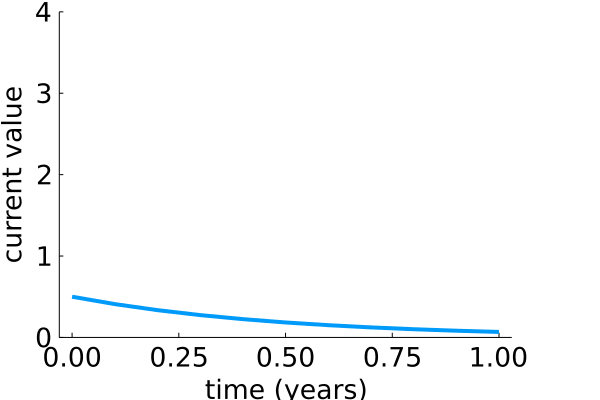# Day_04: Solving differential equations¶

## Foreward note:¶

After ignoring the very obvious advice to install Julia from the official website or repo, I finally uninstalled the Arch package (via pacman -S julia) and the Anaconda package (via conda install -c conda-forge julia) and installed Julia v1.7.2 through

• git clone https://github.com/JuliaLang/julia.git

• cd julia

• make

• ./julia I thought my Arch system would be up-to-date enough for the Julia packages or maybe Jupyter would work better if I used the Anaconda package. All wrong, just follow the well-documented path and install the correct packages. Learn from my mistakes.

Today, I wanted to solve some differential equations. The DifferentalEquations documentation has some great initial examples. I chose the 1D exponential model to start,

$$\frac{du}{dt} = \lambda u$$

where $$\lambda$$ is the exponential growth/decay parameter. For positive values, the more you have, the more you get. For negative values, the more you have, the more you lose.

using Plots, LaTeXStrings
using DifferentialEquations


## Setting up the differential equation¶

In this step, I built the differential equation as a function of u, p, and t. Here the

• u is the state of the differential equation, starting at an initial condition, u0 = 1/2.

• params are the parameters in the function, in this case its my value of $$\lambda$$

• t is the current time

f(u,params,t) = params*u;
u0 = 1/2;
tspan = (0.0,1.0);
p = 1.01;
prob = ODEProblem(f,u0,tspan,p)

ODEProblem with uType Float64 and tType Float64. In-place: false
timespan: (0.0, 1.0)
u0: 0.5


## Solving the differential equation¶

Now, prob is an ODEProblem that contains

• the function, f(u, params, t)

• the initial value (at t = 0), u0

• the timespan, (0, 1.0)

• and the parameters, params = p

Solving this oridinary differential equation is now done with solve as such,

sol = solve(prob)

retcode: Success
Interpolation: specialized 4th order "free" interpolation, specialized 2nd order "free" stiffness-aware interpolation
t: 5-element Vector{Float64}:
0.0
0.09964258706516003
0.3457024247583422
0.6776921908052249
1.0
u: 5-element Vector{Float64}:
0.5
0.552938681151017
0.7089376245893466
0.9913594502399236
1.3728004409033037


## Checking the output¶

The output from solve is really nice. You can

• check the time steps, t: 5-element Vector {Float64}

• check the solutions, u: 5-element Vector {Float64}

• interpolate between values, sol(0.5)

• plus more

For example, here I plot the time vs current value. Then, add the interpolated point at t = 0.5

Plots.theme(:cooper)
halftime = 0.5
plot(sol.t, sol.u, markershape = :utriangle, label = "solutions")
scatter!([halftime], [sol(halftime)], markershape = :square, label = "interpolated")
plot!(xlabel = "years", ylabel = "current value")## Changing parameters in model¶

Next, I wanted to change the value of $$\lambda$$. Below I set up a for-loop to change $$\lambda = 1.1,~ 1.01,~ 0,~ -1,~ -2$$. I have to create a new problem in each case before running the solver and plotting the results. I also create smoother lines by interpolating with 11 time steps.

Note: when I plot inside a for-loop, I needed to create an initial empty plot and assign it to a variable, plts. Then, I added plots inside the loop using plot!. Finally, displaying the result with a call to plts

t = range(0, 1, 11)

plts = plot(bg=:white, xlabel = "time (years)", ylabel = "current value")
for p in [1.1, 1.01, 0, -1, -2]
prob = ODEProblem(f,u0,tspan,p)
sol = solve(prob)
t = range(0, 1, 11)
plot!(t, sol(t), label = string(L"$\lambda$","= $p")) end plts## Animating the effect of change¶ Its amazing what the effect of exponential growth can have on your health, knowledge, bank account, etc. If each day you’re increasing your knowledge by 1%, then by the end of the year you don’t have 100+365% knowledge, you have 3,778% of the knowledge you had a year ago. When you continuously integrate, it grow faster. Here I build an animation from exponential decay, $$\lambda = -2$$ to exponential growth, $$\lambda = 2$$ every 0.1 steps. First, I set up the plot background, labels, and ylimits. Then, use the @gif to loop through each value of $$\lambda$$ for parameter, p. plts = plot(bg=:white, xlabel = "time (years)", ylabel = "current value", ylims = (0, 4)) @gif for p in -2:0.1:2 prob = ODEProblem(f,u0,tspan,p) sol = solve(prob) t = 0:0.1:1 plot!(t, sol(t), label = "")#string(L"$\lambda$","=$p"))
end

┌ Info: Saved animation to
│   fn = /home/ryan/Documents/Career_docs/cooperrc-gh-pages/Julia-learning/tmp.gif
└ @ Plots /home/ryan/.julia/packages/Plots/D9pfj/src/animation.jl:114## Wrapping up¶

Today, I had planned to look at a more advanced set of differential equations, but I ran into Pkg version issues because I did not follow the standard practices that were suggested. I love the “free” interpolation in the DifferentialEquations solver. I have made so many numerical solutions that I solve with unnecessary precision and number of timesteps because I wanted to compare specific values at given times. These first-order growth/decay models converge quickly, but sometimes I need to know a value at $$t=0.6$$, so I resolve the differential equation with smaller steps. It makes much more sense to interpolate.